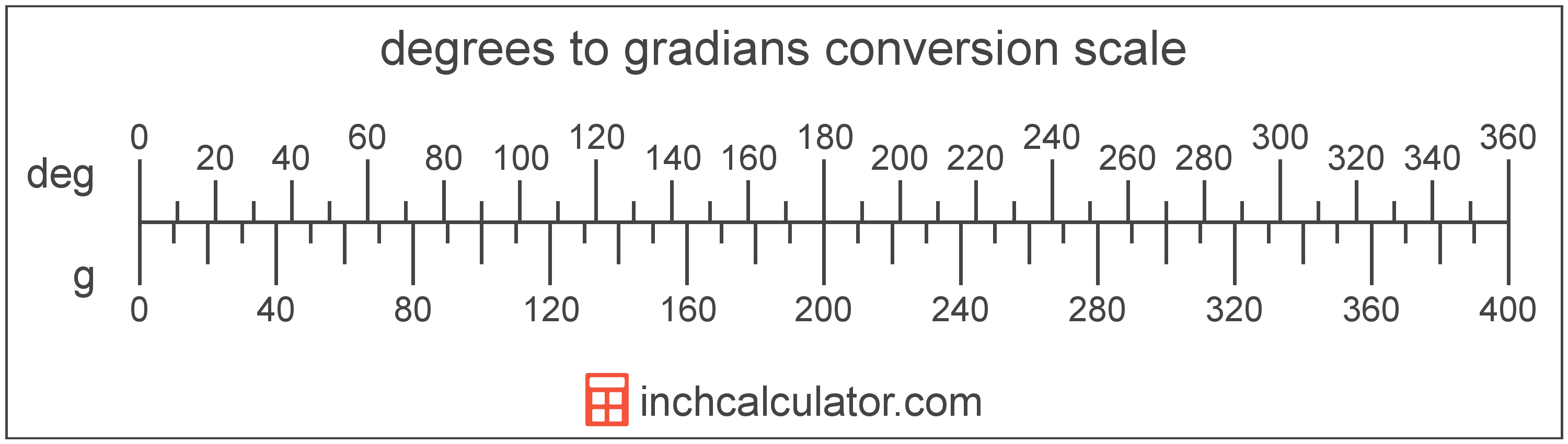Enter the angle in degrees below to get the value converted to gradians.1° = 1.111111g

Do you want to convert gradians to degrees?

## How to Convert Degrees to Gradians

To convert a measurement in degrees to a measurement in gradians, multiply the angle by the following conversion ratio: 1.111111 gradians/degree.

Since one degree is equal to 1.111111 gradians, you can use this simple formula to convert:

The angle in gradians is equal to the angle in degrees multiplied by 1.111111.

For example, here's how to convert 5 degrees to gradians using the formula above.
gradians = (5° × 1.111111) = 5.555556g### How Many Gradians Are in a Degree?

There are 1.111111 gradians in a degree, which is why we use this value in the formula above.

1° = 1.111111g

## What is a Degree?

A degree is a measure of angle equal to 1/360th of a revolution, or circle. The number 360 has 24 divisors, making it a fairly easy number to work with. There are also 360 days in the Persian calendar year, and many theorize that early astronomers used 1 degree per day.

The degree is an SI accepted unit for angle for use with the metric system. A degree is sometimes also referred to as a degree of arc, arc degree, or arcdegree. Degrees can be abbreviated as °, and are also sometimes abbreviated as deg. For example, 1 degree can be written as 1° or 1 deg.

Degrees can also be expressed using arcminutes and arcseconds as an alternative to using the decimal form. Arcminutes and arcseconds are expressed using the prime (′) and double-prime (″) characters, respectively, although a single-quote and double-quote are often used for convenience.

One arcminute is equal to 1/60th of a degree, and one arcsecond is equal to 1/60th of an arcminute.

Protractors are commonly used to measure angles in degrees. They are semi-circle or full-circle devices with degree markings allowing a user to measure an angle in degrees. Learn more about how to use a protractor or download a printable protractor.

A gradian is equal to 1/400 of a revolution or circle, or 9/10°. The grad, or gon, is more precisely defined as π/200, or 1.570796 × 10-2 radians.

This unit simplifies the measurements of right angles, as 90° is equal to 100 gradians.

A gradian is sometimes also referred to as a grad, gon, or grade. Gradians can be abbreviated as g, and are also sometimes abbreviated as gr or grd. For example, 1 gradian can be written as 1g, 1 gr, or 1 grd.

In the expressions of units, the slash, or solidus (/), is used to express a change in one or more units relative to a change in one or more other units.

## Degree to Gradian Conversion Table

Table showing various degree measurements converted to gradians.
1.1111g
2.2222g
3.3333g
4.4444g
5.5556g
6.6667g
7.7778g
8.8889g
10g
10° 11.11g
11° 12.22g
12° 13.33g
13° 14.44g
14° 15.56g
15° 16.67g
16° 17.78g
17° 18.89g
18° 20g
19° 21.11g
20° 22.22g
21° 23.33g
22° 24.44g
23° 25.56g
24° 26.67g
25° 27.78g
26° 28.89g
27° 30g
28° 31.11g
29° 32.22g
30° 33.33g
31° 34.44g
32° 35.56g
33° 36.67g
34° 37.78g
35° 38.89g
36° 40g
37° 41.11g
38° 42.22g
39° 43.33g
40° 44.44g

## References

1. Collins Dictionary, Definition of 'degree', https://www.collinsdictionary.com/us/dictionary/english/degree
2. Ambler Thompson and Barry N. Taylor, Guide for the Use of the International System of Units (SI), National Institute of Standards and Technology, https://physics.nist.gov/cuu/pdf/sp811.pdf